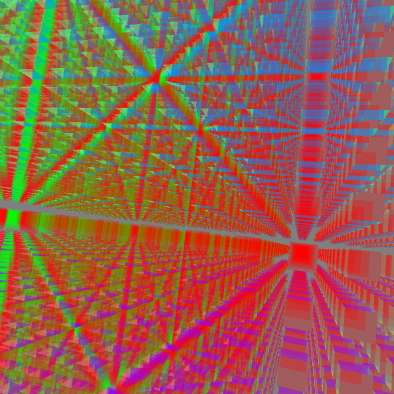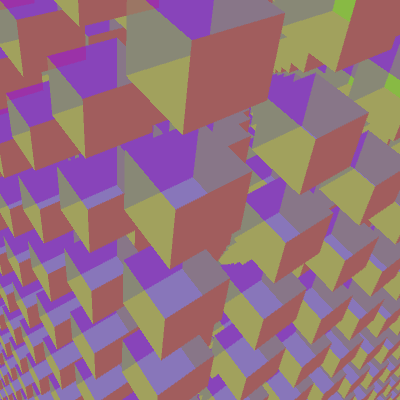#### Howdy, Stranger!

We are about to switch to a new forum software. Until then we have removed the registration on this forum.

# PShape within a Class

edited November 2016

I am trying to create a lot of cubes. If I just put createShape inside my Setup, then I get 8000 references to the same cube, so I want to make 8000 children cubes that I can modify the translation and rotation of each one. I am missing something with the Class setup as I am getting a Type MisMatch.

I would appreciate any insight into what I might be doing wrong. Thanks for the help.

``````import processing.opengl.*;
import peasy.*;
PeasyCam cam;
PShape world;
float CS = .25;
int CPS = 20;
int TotalCubes=CPS*CPS*CPS;
float offset = CPS/2;
int CC = 0;  //CubeCount
PShape tempcube;

void setup()  {
size(2100, 2100, P3D);
cam = new PeasyCam(this, 15000);
noStroke();

println("Making World");
world=createShape(GROUP);
for (int i = 0; i < TotalCubes; i++) {
tempcube = new MyCube();
println(i);          }

println("Translating cubes");
for (int i = 0; i < CPS; i++) {
for (int j = 0; j < CPS; j++) {
for (int k = 0; k < CPS; k++) {
PShape tempcube = world.getChild(CC);
tempcube.translate(i-offset,j-offset,k-offset);
println(CC++, tempcube);     }}}
} // end setup

void draw()  {
background(127);
scale(90);
shape(world,0,0);
//Change_Cells();
} // end draw

class MyCube {
PShape output;

MyCube() {
int size = 1;
println("Making CUBE");
PShape output = createShape();
output.fill(0x44FF0000); output.vertex(-CS,  CS,  CS);output.vertex( CS,  CS,  CS);output.vertex( CS, -CS,  CS);output.vertex(-CS, -CS,  CS);
output.fill(0x4400FF00); output.vertex( CS,  CS,  CS);output.vertex( CS,  CS, -CS);output.vertex( CS, -CS, -CS);output.vertex( CS, -CS,  CS);
output.fill(0x440000FF); output.vertex( CS,  CS, -CS);output.vertex(-CS,  CS, -CS);output.vertex(-CS, -CS, -CS);output.vertex( CS, -CS, -CS);
output.fill(0x44FFFF00); output.vertex(-CS,  CS, -CS);output.vertex(-CS,  CS,  CS);output.vertex(-CS, -CS,  CS);output.vertex(-CS, -CS, -CS);
output.fill(0x4400FFFF); output.vertex(-CS,  CS, -CS);output.vertex( CS,  CS, -CS);output.vertex( CS,  CS,  CS);output.vertex(-CS,  CS,  CS);
output.fill(0x44FF00FF); output.vertex(-CS, -CS, -CS);output.vertex( CS, -CS, -CS);output.vertex( CS, -CS,  CS);output.vertex(-CS, -CS,  CS);
output.endShape(CLOSE);
return output;
} // end constructor
}// end MyCube
``````
Tagged:

• In your `MyCube` class, you have a class-level `output` variable. Then in your `MyCube()` constructor, you have a different variable named `output` that hides your class-level `output` variable. If you want to reference the class-level variable, don't declare it again in your constructor.

• I am confused. So If I just want the class to create a new instance of the cube each time, I need to get rid of line 42 or co I need to replace 42 with line 47? How does either of these give me the Type Mismatch on line 21 when I try to call it?

• grrr

8000 lines of println output is going to break something

``````println(CC++, tempcube);
``````

hiding an increment inside a seeming safe instruction like a println is a terrible idea. try and be clearer about stuff.

• edited November 2016 Answer ✓

ok, slight rewrite - the create and the translate now done at the same time - passing offset into the constructor.

reduced the debug output.

resized the screen so it wasn't 10 times larger than my laptop... 8)

``````import processing.opengl.*;
import peasy.*;

PeasyCam cam;
PShape world;
float CS = .25;
int CPS = 20;
float offset = CPS/2;

void setup() {
size(1024, 640, P3D);
cam = new PeasyCam(this, 1500);
noStroke();

println("Making World");
world = createShape(GROUP);
println("Translating cubes");
for (int i = 0; i < CPS; i++) {
for (int j = 0; j < CPS; j++) {
for (int k = 0; k < CPS; k++) {
MyCube cube = new MyCube(i - offset, j - offset, k - offset);
world.addChild(cube.output); // <- nb not MyCube, needs to be the PShape
}
}
}
println("done");
} // end setup

void draw() {
background(127);
scale(90);
shape(world, 0, 0);
//Change_Cells();
} // end draw

// could just be a method, returning PShape
class MyCube {
PShape output;

MyCube(float x, float y, float z) { // <- new arguments
int size = 1;
output = createShape();
output.translate(x, y, z); // <- new tanslate
output.fill(0x44FF0000);
output.vertex(-CS, CS, CS);
output.vertex( CS, CS, CS);
output.vertex( CS, -CS, CS);
output.vertex(-CS, -CS, CS);
output.fill(0x4400FF00);
output.vertex( CS, CS, CS);
output.vertex( CS, CS, -CS);
output.vertex( CS, -CS, -CS);
output.vertex( CS, -CS, CS);
output.fill(0x440000FF);
output.vertex( CS, CS, -CS);
output.vertex(-CS, CS, -CS);
output.vertex(-CS, -CS, -CS);
output.vertex( CS, -CS, -CS);
output.fill(0x44FFFF00);
output.vertex(-CS, CS, -CS);
output.vertex(-CS, CS, CS);
output.vertex(-CS, -CS, CS);
output.vertex(-CS, -CS, -CS);
output.fill(0x4400FFFF);
output.vertex(-CS, CS, -CS);
output.vertex( CS, CS, -CS);
output.vertex( CS, CS, CS);
output.vertex(-CS, CS, CS);
output.fill(0x44FF00FF);
output.vertex(-CS, -CS, -CS);
output.vertex( CS, -CS, -CS);
output.vertex( CS, -CS, CS);
output.vertex(-CS, -CS, CS);
output.endShape(CLOSE);
} // end constructor
}// end MyCube
``````
• Nice. Counting them up, that looks like exactly.... 8000 cubes.• and it moves nicely. i'm not sure the transparency is perfect though - that looks like it is depth testing so it's not drawing cubes behind the visible ones even though it should be.

to do it properly you'd need to disable depth testing and sort them so they are drawn furthest first. which is a bunch of work.

• THANK YOU both very much. I am learning and I appreciate the help. I will use this and start from her with my next part. FYI, the reason for the transparency is that I need to run different scenarios and be able to visually see if/how the cubes are changing. If they are not transparent, it is harder to see the effect.

Again, THANK YOU